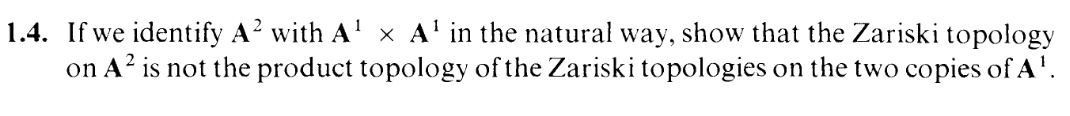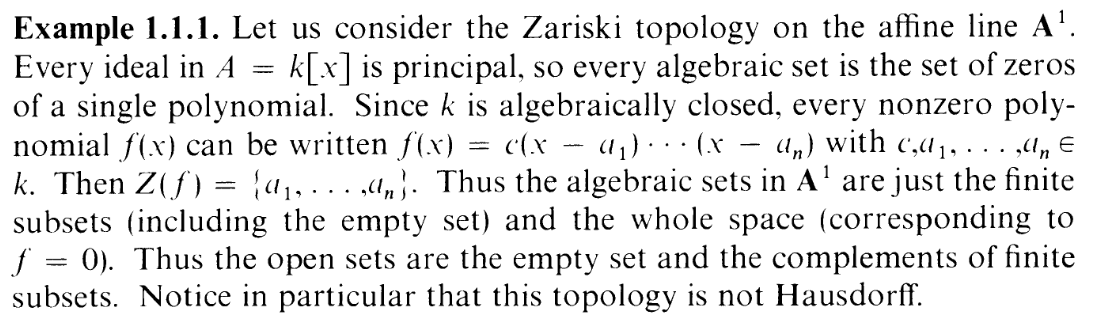I.1.4

11/30/2020It looks like this time we've got a topological exercise rather than an algebraic one? Actually, the whole "point" of the Nullstellensatz appears to be a duality between algebraic sets (rings) and topological spaces (An), so can someone explain to me why this subject is called "algebraic geometry" rather than "algebraic topology"? I mean, I know the latter name is already reserved for another branch. But where's the "geometry" in all this? Is it because the spaces we're looking at are the zero sets of polynomials? That's geometry?

Lol, whatever. Wait. WAIT. I guess this exercise demonstrates exactly what I was talking about? We're comparing the "topological" definition of A2 (i.e. using the product topology) with the "geometric" definition of A2 (i.e. using the Zariski topology–the zero sets of polynomials). Am I right here, or am I just flailing around like an idiot.

Well, anyway, here we go. The Zariski topology of A1 has already been expained in the book:I.e. the open sets of A1 fall into the following classes
1)
2) complements of finite sets
3) A1

So a base for the product topology of A2 is (S an arbitrary complement of a finite set):
1)
2) S × A1
3) A1 × S
4) S1 × S2
5) A2

Well, "complements of finite sets" are annoying to think about. Let's make a closed set base instead, by complementing all of these.

We get the following closed set base for the product topology of A2 (F an arbitrary finite set):
1) A2 (the whole space)
2) A1 × F (finitely many horizontal lines)
3) F × A1 (finitely many vertical lines)
4) F1 × F2 ("a finite set of points")
5) (the empty set)

A closed set base generates the topology by taking intersections of the base elements. Clearly (using this theorem) intersecting any of these classes of sets gives us another set in the class. So the base above is actually a complete description of the topology (wtf? kind of suspicious but ok).

So, obviously Y = Z(y2 -x2 - 1) (the unit circle) is closed in the Zariski topology of A2 but isn't a closed set in the product topology. So the spaces are not homeomorphic. (Though I believe the Zariski topology is finer than the product topology? Don't quote me on that though lol)